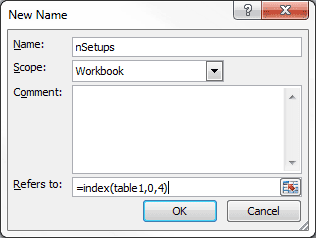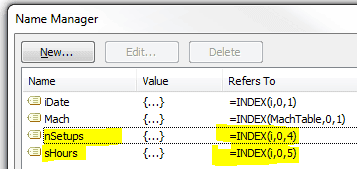# Dynamic Table Reference with INDEX

Tables have structured data so you can easily use column references in formulas. But with the Table name AND column name, the reference can be rather lengthy and hard to understand at first glance.

As an alternative, you can simply create a dynamic column reference in a Table by using the INDEX function in a Defined Name.

First I will explain how the INDEX function works to capture a dynamic Table range, then I’ll create a Defined Name using the INDEX function, use it in a formula, and see what happens when things change.

## The Dynamic INDEX

The syntax is INDEX(array, row_num, [column_num]) where array refers to the Table name, the row_num reference is set to zero (0), and the column_num is a number of the Table column you want to reference.

Using zero (0) for the row_num argument forces all rows in the column to be returned.

## Create a Defined Name Formula with INDEX

I’m going to use an example for the column Number Setups in Table1.

In Excel 2010 and 2007 (Windows) choose Formulas > Name Manager and click New. In the New Name dialog box type nSetup. In the Refers to: box type the formula =INDEX(Table1,0,4) then click OK. You can also omit the zero for an equivalent formula =INDEX(Table1,,4).This next example will use the Table1 column Setup Hours. In Excel 2011 (Mac) choose Insert > Name > Define. In the Define Name dialog box type sHours into the Names in workbook box. In the Refers to: box type the formula =INDEX(Table,1,5) then click OK.

I have an existing formula for Average Hours per Setup using structured Table references that looks like this:

=SUBTOTAL(109,Table1[Setup Hours])/SUBTOTAL(109,Table1[Number Setups])

Using the Defined Names we create a formula like this:

=SUBTOTAL(109,sHours)/SUBTOTAL(109,nSetups)

Both formulas are equivalent, but the second is shorter and a bit more clear (in a techno-geek kinda way).

## Changes to Be Aware Of

If you change the name of the Table, Excel will modify everything related to the name so there are no worries about the Define Name formula. I changed the Table name to i and here’s what the Defined Names look like.If I move the Table to some other place in the worksheet, there’s no effect to the Defined Name formulas because the column references are for the Table and not the worksheet.

However, if you move the column location within the Table, the Defined Name will not change its reference to the new column position. The column reference has to be manually changed in the INDEX function within the Defined Name.

## Conclusion

The moral of this story is that Defined Names using the INDEX function to return a dynamic range reference in a Table can be used to make formulas easier to read, but moving a column within a Table can invalidate the reference.